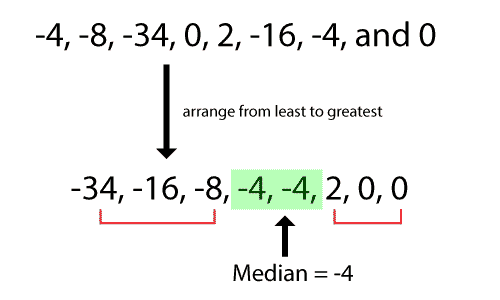# 5 Number Summary Calculator

Enter Information

#### Results

Fill the calculator form and click on Calculate button to get result here

Get 5 Number Summary Calculator For Your Website

The 5 number summary calculator is used to calculate the five number summary, which consists of five different calculations. These calculations include:

• Minimum number
• The first quartile Q1
• Median
• The second quartile Q2, and
• Maximum number

All these calculations are very useful because it provides a summary of the range of our data. It gives information about the range, location, and spread of the data. In this post, we will explain how to use our five number summary calculator, how to calculate 5 number summary, and much more.

## How to use 5 number summary calculator?

Five number summary calculator makes it very easy to calculate all five values on one click. You don’t have to calculate each of the values separately because this calculator provides every value once you click the calculate button. However, if you want to calculate quartiles, you can use our quartile calculator anytime.

To fuse this calculator, follow the below steps:

• Enter your values in the given input box.
• Separate each number using a comma.
• Press the Calculate button to see the five number summary of entered numbers.

It will give you the minimum number, maximum number, first quartile, third quartile, and median as soon as you hit the button. Moreover, it will calculate interquartile, ascending order, and descending order too.

The 5 number summary calculator also produces a graph based on the entered values by the user. This graphical representation of the five number summary is very helpful to understand the spread, range, and location of data. Hover of the graph to see how your values are distributed on the graph.

## What are the steps to find 5 number summary?

As we have described earlier, 5 number summary consists of a total of five values, which are minimum and maximum numbers, median, and first and third quartile. Follow these steps to calculate the five number summary.

1. Arrange your data in ascending order (from lower to higher).
2. Get the minimum and maximum values from the arranged values.
3. Find the median from these values. Median can be found by locating the middle value in an arranged set of data. If there are two middle numbers, add them and divide them with 2 to get the median.4. Wrap the parenthesis around the values which are on the left and right side of the median. See the below image.5. Find the first and third quartiles. 1st quartile can be found as the median in the lower quartile, and 3rd quartile can be found as the median in the upper quartile.
6. Write down all values together to get a summary.

### Example:

Let’s calculate 5 number summary using an example to understand the process properly.

Calculate the five number summary in data set 5, 2, 19, 6, 7, 1, 18, 9, 12, 15, 27.

Solution:

Step 1: Arrange the data set in ascending order.

1, 2, 5, 6, 7, 9, 12, 15, 18, 19, 27

Step 2: Get the minimum and maximum values in the data set.

Minimum value = 1

Maximum value = 27

Step 3: Find the median from these values. Median can be found by locating the middle value in an arranged set of data. If there are two middle numbers, add them and divide them with 2 to get the median.

Median = 9

Step 4: Wrap the parenthesis around the values which are on the left and right side of the median.

(1, 2, 5, 6, 7), `9`, (12, 15, 18, 19, 27)

Step 5: Find the first and third quartiles. 1st quartile can be found as the median in the lower quartile, and 3rd quartile can be found as the median in the upper quartile.

Q1 = 5

Q2 = 18

Step 6: Write down all values together to get a summary.

1. Minimum = 1
2. Q1 = 5
3. Median = 9
4. Q3 = 18
5. Maximum = 27
Other Languages
##### Not Available for 5 Number Summary Calculator
Related Calculators